### The semantics of B

Previously we introduced the B language.

We were left wondering how to work out what our evaluator should do, and how we should determine if it is behaving correctly.

It’s time to bring some PLT into the mix.

# Values

A subset of our terms will be values.

A value is a term that cannot be evaluated any further - that’s what it means to be a value, hence the name evaluator.

The evaluator should be able to transform any term in our language into a value.

For the language B, the values can be defined as:We can also define them in natural deduction style.If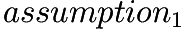and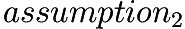hold, then we can conclude that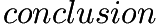holds. We also say that we can derivefromand.

We’re defining the judgment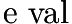, such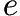is a value if we can derive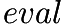from the below rules. In this case things are pretty simple, since we don’t have any assumptions.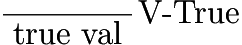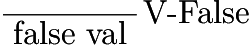We could write a test for whether a term is a value:

isValue :: Term
-> Bool
isValue TmTrue  =
True
isValue TmFalse =
True
isValue _       =
False

We’re going to take a different path which will simplify the testing and the composability of our system. It’s may look like a lot of unnecessary complexity, but the reasons for these changes will soon be made clear.

We define the function value:

value :: Term
-> Maybe Term
value TmTrue  =
Just TmTrue
value TmFalse =
Just TmFalse
value _       =
Nothing

which returns Nothing for non-values, from which we can define isValue:

isValue :: Term
-> Bool
isValue =
isJust .
value

We can break value up, so that we have a function for each of the rules. The functions will use Maybe, to handle the case where the rule doesn’t hold.

Sobecomes

valueTmTrue :: Term
-> Maybe Term
valueTmTrue TmTrue =
Just TmTrue
valueTmTrue _ =
Nothing

andbecomes

valueTmFalse :: Term
-> Maybe Term
valueTmFalse TmFalse =
Just TmFalse
valueTmFalse _ =
Nothing

Now we need to combine them back together, which we can do with the Alternative instance for Maybe, which chooses the first of these that return something other than Nothing:

value :: Term
-> Maybe Term
value tm =
valueTmTrue tm <|>
valueTmFalse tm 

This will mean that the order will matter if there is overlap between the rules. That’s a concern we don’t really want to have, but we can take care of that elsewhere.

The use of the Alternative instance can be generalized to a list of rules, by using asum from Data.Foldable:

asum :: (Foldable t, Alternative f)
=> t (f a)
-> f a

to get:

value :: Term
-> Maybe Term
value tm =
asum
[ valueTmTrue tm
, valueTmFalse tm
]

We can simplify that:

value :: Term
-> Maybe Term
value tm =
asum .
map ($tm)$
[ valueTmTrue
, valueTmFalse
]

and then we can separate out the rules from the value function:

valueRules :: [Term -> Maybe Term]
valueRules =
[ valueTmTrue
, valueTmFalse
]

value :: Term
-> Maybe Term
value tm =
asum .
map ($tm)$
valueRules

That is a bit of jump from isValue, but I promise you it’ll lead somewhere interesting.

# Small-step semantics

We now need a systematic way to turn a term into a value.

We do this by defining a single step of evaluation - a single change that moves a term closer to being a value. We denote a step from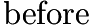to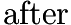as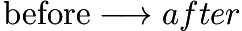The idea for B is that if we have a set of rules for the single steps of evaluation and the rules cover all of the non-value terms then we’ll always be able to do something. If either none of the rules overlap, or if the set of rules that apply for a given term all give the same result, then our rules will be deterministic.

We refer to a term for which there is no applicable rule as a normal form, and we refer to the process of repeatedly running these rules until no rules apply as normalization.

Every value is a normal form.

For B every normal form is a value, although this won’t always be the case. We’ll refer to any term that is normal form and is not a value as stuck.

If we don’t have any stuck terms, then evaluation and normalization are going to be more or less the same thing.

This is a pretty nice place to be in. The values are defined in relation to the syntax - the structure of the terms - and the normal forms are defined in terms of the semantics - via the small-step relation - so having having agreement between those two concepts means we’re on the right track.

## Small-step semantics for B

Since we have already designated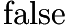and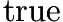as values, we only need to deal with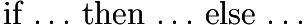in our rules.

We have three cases to consider.

When we’re dealing with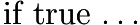, we step to the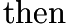case: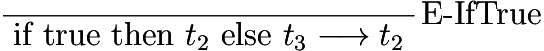When we’re dealing with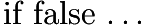, we step to the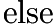case: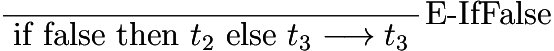We’ve now dealt with all of the cases of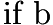where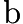is a value, so we now deal with the case wherecan take a step:The combination of these three rules define the small-step relation for B.

It’s worth noting that the rules are exhaustive (all terms in B will match at least one of these rules) and disjoint (all terms in B will match at most one of these rules).

The first two rules are referred to as reduction rules, and the third rule is known as a congruence rule. The reduction rules do the computation, and the congruence rules control the order of evaulation and whether the evaluation is lazy or not.

We’ll convert these into Haskell so that we can play with them. There will be one function per rule, just like we had with the values.

The first two rules are straightforward.becomes:

eIfTrue :: Term
-> Maybe Term
eIfTrue (TmIf TmTrue t2 _) =
Just t2
eIfTrue _                  =
Nothing

andbecomes:

eIfFalse :: Term
-> Maybe Term
eIfFalse (TmIf TmFalse _ t3) =
Just t3
eIfFalse _                   =
Nothing

The last case is recursive:and becomes:

eIf :: (Term -> Maybe Term)
-> Term
-> Maybe Term
eIf step (TmIf t1 t2 t3) = do
t1' <- step t1
return $TmIf t1' t2 t3 eIf _ _ = Nothing We pass the step function into the rule so that we can worry about the recursion later. The step function works in the Maybe monad since it isn’t defined for all terms. At the moment it isn’t defined for the values - and it shouldn’t be, since values are meant to be normal forms - but soon we’ll have a language where non-value terms may not have rules that apply. In the general case for our natural deduction rules, we’ll be working in a monad with a notion of failure, and this failure will encompass the pattern match failures for our rules. We can now combine these rules into a smallStep function, in the same way that we combined the rules for values into the value function. smallStepRules :: [Term -> Maybe Term] smallStepRules = [ eIfFalse , eIfTrue , eIf smallStep ] smallStep :: Term -> Maybe Term smallStep tm = asum . map ($ tm) $smallStepRules The difference between this and what we did with values is that the smallStepRules and smallStep function are mutually recursive. This extra level of indirection means we can define groups of rules in different modules, and then import them and add them to smallStepRules when we want to use them. That is a nice step towards the incremental definitions of languages that appear in PLT literature, and is the reason value took the form that it did. Now that we have smallStep, we can define isNormalForm :: Term -> Bool isNormalForm = isNothing . smallStep in a similar manner to how we defined isValue earlier on. We could also define isStuck :: Term -> Bool isStuck t = not (isNormalForm t || isValue t) ## Evaluation We can now build an evaluator from the small step function. As we’re using the small-step semantics to define the meaning of evaluation, if we implement the evaluation rules correctly we can be pretty confident in the results. It may not be all that fast - since it is doing some redundant pattern matching - but it’s still useful. Technically, our evaluation function is the reflexive transitive closure of smallStep. We’re going to transform terms into terms: eval :: Term -> Term eval tm =  by taking a step:  case step tm of If we can’t take a step, the term is a value, so we’re done:  Nothing -> tm and if we can take a step, we recurse to keep going:  Just tm' -> eval tm' All together: eval :: Term -> Term eval tm = case smallStep tm of Nothing -> tm Just tm' -> eval tm' # Aside: Why the weird implementations of value and smallStep? I’m working towards something here, but I want to get a lot of pieces in place first. It’s probably worth saying at least a little bit about the direction I’m heading so that not too many people fall asleep or wander off. If you’ve watched the talk, you probably already know this is on the roadmap, although I rushed through a lot of the details. As a first, let’s introduce another layer of indirection. We’ll introduce data structures for these rules: data ValueRule tm = ValueBase (tm -> Maybe tm) data SmallStepRule tm = SmallStepBase (tm -> Maybe tm) | SmallStepRecurse ((tm -> Maybe tm) -> tm -> Maybe tm) and we’ll combine the rules into another data structure: data TermRules tm = TermRules { tmValueRules :: [ValueRule tm] , tmSmallStepRules :: [SmallStepRule tm] } which has a monoid instance: instance Monoid (TermRules tm) where mempty = TermRules mempty mempty mappend tm1 tm2 = TermRules (mappend (tmValueRules tm1) (tmValueRules tm2)) (mappend (tmSmallStepRules tm1) (tmSmallStepRules tm2)) The plan is that these data structures will grow to include type inference, test data generation, parsing and printing. They’ll go into a package - let’s call it language-input. We’ll write another package - say, language-output - that combines these rules together in order to create the value, smallStep and eval functions that we have already seen, as well as parsers and printers for terms, all the way through to creating test suites and a REPL. We can then write various packages that gather up the rules for various language fragments, which would just depend on language-input. Once we have all of those, we can do things like: repl :: IO () repl = mkRepl$ booleanRules <> natRules <> stlcRules

The various pieces I’m going to introduce along the way will alter how all of this looks, so I’m going to talk about this in one place once everything is position and ready to go.

Until then, try to keep this goal in mind - the extra bits of complexity are there for a reason. I hope the payoff is worth the wait.

# Theorems

Back in the here and now, we have more immediate concerns - we still could have messed up our rules. The patterns could overlap, we could have forgotten something, or there could be a typo in our code. We want to try to rule that out.

We do this by coming up with behaviours and properties that we think should be reasonable, and then demonstrating that they are true. For instance, it’d be nice to know that our eval function always terminates. Failing that, it would be nice to understand the circumstances under which it fails to terminate.

In the PLT space, the work of proposing and checking properties is done by coming up with theorems and proving them. These theorems are usually phrased in such a way that they will hold across a useful subset of languages that people are interested in. We can then prove those theorems hold in our language, often using proof techniques that are made familiar to us when we learn and start using those theorems.

For now we have a few properties of interest.

• Every value is a normal form

This means that if a term is a value, then it won’t take a step.

• B has no stuck terms

There is a double negative to unpack here (take that, constructivists). Once the dust settles, this is claiming that if a term is not a value, it will take a step.

• B is determinate

This means that for a given term that can take a step, we will always get the same result from the step function.

We most get this for free from the fact that smallStep is a pure function. This is partly because we are combining the rules in a fixed order, which could be masking issues here.

We can make sure that we’re not missing anything by testing that exactly one small-step rules applies for each non-value term. Later, if we need to handle the case where multiple rules apply for certain terms, we will need to check that the results of those rules always agree for those terms.

• B is normalizing

This means that for any term we care to feed to the evaluator, it will always terminate.

The usual terminology is ‘strongly normalising’ (regardless of evaluation order, normalisation terminates) or ‘weakly normalising’ (there is at least one evaluation order for which normalisation terminates).

If we’re writing tests we could do this computationally, at the risk that our tests might hang or take a long time to run. Using the existing theory lets us do better than that.

We can demonstrate that normalisation of B terminates by showing that, whenever the smallStep function returns a non-Nothing value, the output term is smaller than the input term. If our terms have finite size then a finite number of calls to smallStep will reduce them to a size of zero or a normal form, and that’s when our evaluator returns.

# Aside: Big-step semantics

There’s an alternative way of specifying the operational semantics of a language, called big-step semantics.

Big-step semantics is defined in terms of the evaluation function, which takes terms the whole way to values immediately, rather than in a series of steps.

We use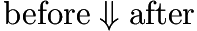to indicate thatevaluates to.

Since the evaluation function maps values to values, we end up not making a distinction between rules for values and rules for steps.

For B we have four rules.

There are two rules to handle what used to be the values: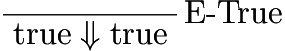and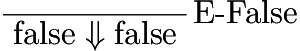There are two rules for the branches of the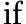expression:andAll that you really need to know at the moment is that we should also be able to evaluate a term using the small step evaluator and the big step evaluator and get the same results.

There are some PLT theorems that we’ll cover later which relate types and semantics, an they all use the small-step semantics. This is at least partly related to the fact that the idea of a well-typed term is closely related to the notion of stuck-ness, and stuck-ness is easier to express and work with when your values are defined separately from your evaluation rules.

I’m including the big-step semantics here because it’s handy to know about.

The big-step semantics also appear in the associated code repository, because testing the two evaluators against each other is really useful.

This is usually done to test a known-to-be-correct evaluator against an evaluator that is faster, or operates over a network, or has otherwise wandered away from the path of obvious-correctness to achieve some other goal. In our case, the evaluator built from the small-step semantics is going to be do a lot of redundant pattern matching, so it’s primary reason for being is to check more efficient evaluators.

It also happens that if I’ve gotten something wrong in the semantics, I usually get it wrong in only one of the small-step or big-step styles, and I like to find out about my errors as soon as I can.

# We have something to prove

If you’ve read HTPI and TAPL then you might have your pen and paper out already and be scribbling away. If you’ve been through Software Foundations, you might be typing away in Proof General.

Otherwise you might be scratching your head a little. Here’s the thing - we’re not going to actually prove these theorems. Instead, we’re going to go for the easiest path we can find to high confidence that these theorems hold.

To begin with, that will involve using QuickCheck, which is the topic of the next post.

• Write the QuickCheck code for the properties that have been mentioned so far.